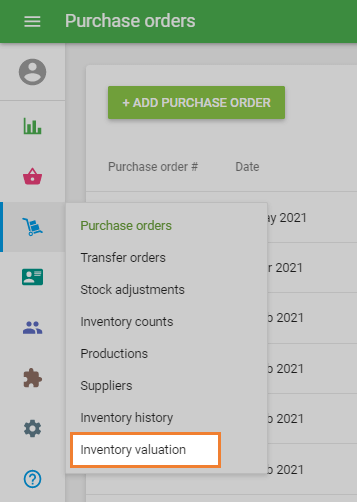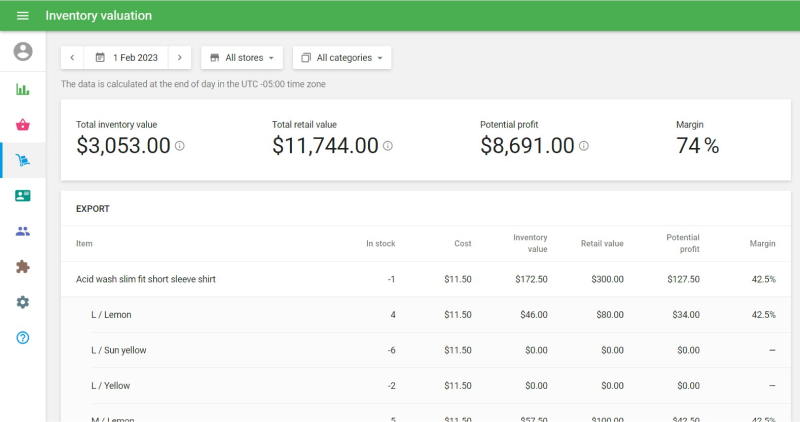# What is Inventory Valuation Report

Topics

Inventory Valuation Report is a part of the advanced inventory management and is only available to those users who have subscribed to this functionality.

Inventory Valuation Report gives an understanding of the total cost of the inventory and potential profits from their sale.

Go to ‘Inventory valuation’ section in the ‘Inventory management’ menu.You can see the up-to-date ‘Inventory valuation’ report. You can filter the report by categories or stores (if you have multiple stores) and export the data by clicking on the ‘Export’ button.There is a summary of the inventory information at the top.
Total inventory value is calculated as the sum of the cost multiplied by the stock of all items.
Total retail value is calculated as the sum of the price multiplied by the stock of all items.
Potential profit is calculated as the difference between the total retail value and total inventory value.
Margin is calculated as Potential profit divided by the Total retail value in percentage.

Note:
Items with a negative stock are not calculated in the Total inventory value.
Items with a negative stock or a variable price (blank price field)  are not calculated in Total retail value and Potential Profit.

You can also see the detailed inventory information of each item.